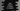# Java program to check if a number is a special number or not## Java program to check if a number is a special number or not:

How to check if a number is a special number or not in Java. We will learn what is a special number and how to check for a special number in Java in different ways.

### Special number:

A number is called a special number if the sum of the factorials of the digits of the number is equal to the number itself. For example, 145 is a special number because the sum of the factorials of the digits of this number is:

``````1! + 4! + 5!
= 1 + 24 + 120
= 145``````

i.e. it is equal to the number itself.

### Algorithm to check for special numbers:

We can use the below algorithm to check if a number is a special number or not:

• Take the number as input from the user.
• Initialize one variable as 0 to hold the sum.
• Iterate over the digits of the number one by one.
• For each digit, find the factorial. To find the factorial,
• Multiply all numbers from 2 to that number. It will be the factorial of the number.
• Add the factorial of each digit to the sum variable.
• Once the factorials of all digits are calculated, the sum variable can be compared to the original number to check if it is a special number or not. If both are equal, it is a special number, else not.

### Example 1: Java program to check if a number is a special number:

Let’s write down the java program to check if a number is a special number or not:

``````import java.util.Scanner;

public class Main {
public static void main(String[] args) {
int number, sum = 0, lastDigit, factorial;
Scanner sc = new Scanner(System.in);

System.out.println("Enter a number: ");
number = sc.nextInt();

int copyNumber = number;
while (copyNumber > 0) {
lastDigit = copyNumber % 10;

factorial = 1;
for (int i = 2; i <= lastDigit; i++) {
factorial *= i;
}

sum += factorial;
copyNumber /= 10;
}

if (number == sum) {
System.out.println(number + " is a special number.");
} else {
System.out.println(number + " is not a special number.");
}
}
}``````

In this program,

• The integer variable number is used to hold the user input number, sum is used to hold the sum of factorials of the digits of the number, lastDigit is used to hold the current last digit of the number while iterating over it and factorial is used to store the current factorial.
• It uses a scanner object sc to read the user input number.
• It creates a copy of the user input number and assigns it to the copyNumber variable.
• The while loop is used to iterate over the digits of the number copyNumber.
• On each iteration of this loop,
• It finds the last digit of the number by using % 10.
• It uses the for loop to find the factorial of the last digit. This value is stored in the factorial variable.
• The calculated factorial is added to the sum variable and the last digit is removed from the copyNumber.
• Once the sum is calculated, it is compared to the original number. If both are equal, it is a special number, else it is not.

If you run this program, it will print outputs as below:

``````Enter a number:
145
145 is a special number.

Enter a number:
133
133 is not a special number.``````

### Example 2: Java program to check if a number is a special number using separate functions:

If we use separate functions, it will make the code clean and readable. Let’s break the above program into different functions:

• One function to check if a number is special or not. It will take one number as its argument and return one boolean value, True if the provided number is a special number and False otherwise.
• Another function to calculate the factorial of a number. This function will be called from the above function. It will also take one number as its argument and return the factorial value for that number.

Let’s write down the program with separate functions:

``````import java.util.Scanner;

public class Main {
private static int getFactorial(int number) {
int factorial = 1;
for (int i = 2; i <= number; i++) {
factorial *= i;
}
return factorial;
}

private static boolean isSpecial(int number) {
int copyNumber = number;
int sum = 0, lastDigit;
while (copyNumber > 0) {
lastDigit = copyNumber % 10;

sum += getFactorial(lastDigit);
copyNumber /= 10;
}
return number == sum;
}

public static void main(String[] args) {
int number;
Scanner sc = new Scanner(System.in);

System.out.println("Enter a number: ");
number = sc.nextInt();

if (isSpecial(number)) {
System.out.println(number + " is a special number.");
} else {
System.out.println(number + " is not a special number.");
}
}
}``````

You can see that this is the same program with two new functions. The getFactorial function is used to find the factorial of a number and isSpecial is used to check if a number is a special number or not.

The main function reads the user input number and calls isSpecial to check if it is a special number or not. The isSpecial function finds the sum of factorial of all the digits. It calls the getFactorial function to calculate the factorial. The isSpecial function returns one boolean value.